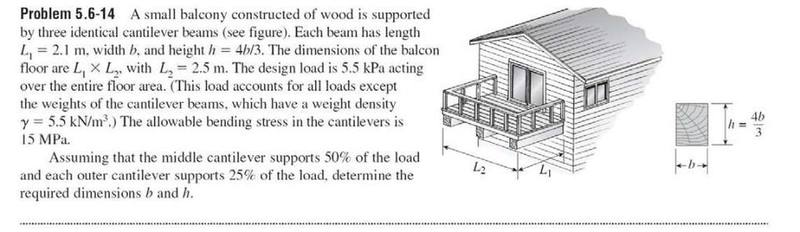# Finding dimensions of a beam using shear, moment, and moment of Inertia

• haleystew

## Homework Statement## Homework Equations

Moment of Inertia equation: I=(1/12)bh^3
Stress and Strain equations: Sigma= -(M*c)/I Tao = (V*Q)/(I*t)

## The Attempt at a Solution

I know I need to use shear and moment diagrams to find the max moment so I can use the Stress diagram to find the Moment of Inertia to find the dimensions of the cross section, but I can't figure out how to get started. Any help would be great. Thank you.

Wouldn't you want to start by setting sigma in your equation equal to the given allowable bending stress? Give it a try.

Can you draw a loading diagram for the cantilever you think has the most stress? Do you know a definition of bending moment that will enable you to draw the bending moment diagram?

I am using the weight density to find the weight distribution over the entire area and then treating that as a simply supported beam. Will this work?

If it is a simply supported beam, rather than a cantilever (as it says in the question !) where are the two supports?